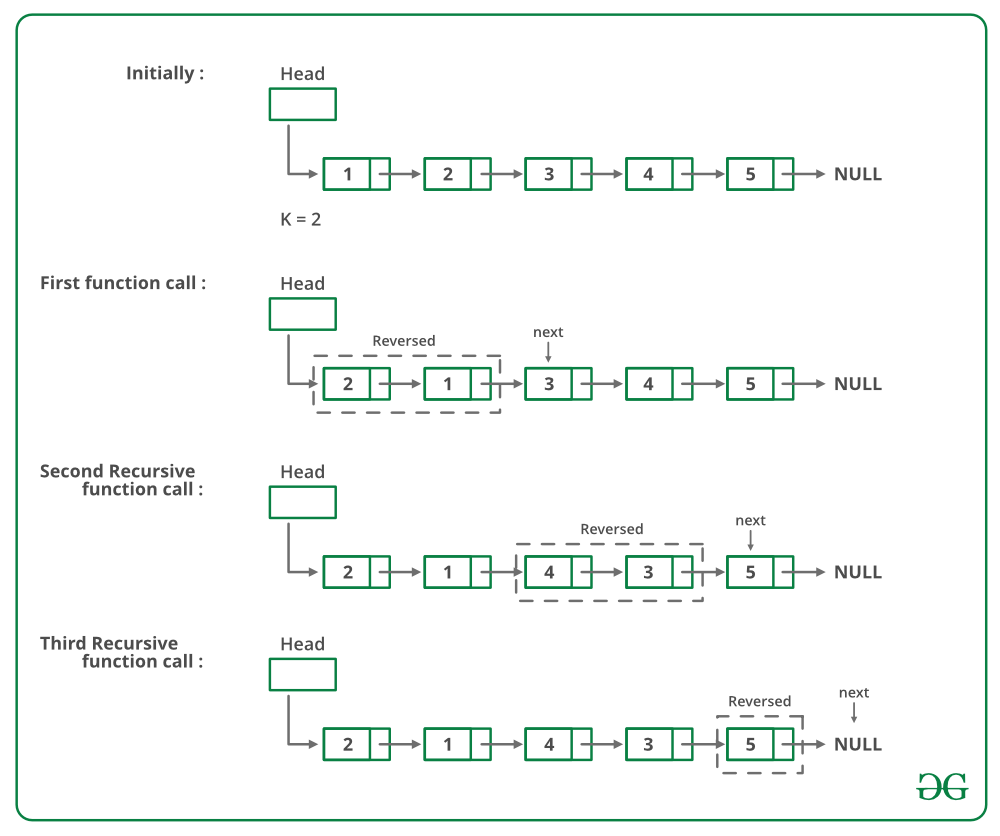# C++ Program For Reversing A Linked List In Groups Of Given Size – Set 1

• Last Updated : 16 Dec, 2021

Given a linked list, write a function to reverse every k nodes (where k is an input to the function).

Example:

Input: 1->2->3->4->5->6->7->8->NULL, K = 3
Output: 3->2->1->6->5->4->8->7->NULL
Input: 1->2->3->4->5->6->7->8->NULL, K = 5
Output: 5->4->3->2->1->8->7->6->NULL

• Reverse the first sub-list of size k. While reversing keep track of the next node and previous node. Let the pointer to the next node be next and pointer to the previous node be prev. See this post for reversing a linked list.
• head->next = reverse(next, k) ( Recursively call for rest of the list and link the two sub-lists )
• Return prev ( prev becomes the new head of the list (see the diagrams of an iterative method of this post )

Below is image shows how the reverse function works:Below is the implementation of the above approach:

## C++

 `// C++ program to reverse a linked list``// in groups of given size``#include ``using` `namespace` `std;`` ` `// Link list node ``class` `Node ``{``    ``public``:``    ``int` `data;``    ``Node* next;``};`` ` `/* Reverses the linked list in groups``  ``of size k and returns the pointer``  ``to the new head node. */``Node* reverse(Node* head, ``int` `k)``{``    ``// Base case``    ``if` `(!head)``        ``return` `NULL;``    ``Node* current = head;``    ``Node* next = NULL;``    ``Node* prev = NULL;``    ``int` `count = 0;`` ` `    ``// Reverse first k nodes of the``    ``// linked list ``    ``while` `(current != NULL && ``           ``count < k)  ``    ``{``        ``next = current->next;``        ``current->next = prev;``        ``prev = current;``        ``current = next;``        ``count++;``    ``}`` ` `    ``/* next is now a pointer to (k+1)th node``       ``Recursively call for the list starting  ``       ``from current. And make rest of the list ``       ``as next of first node */``    ``if` `(next != NULL)``        ``head->next = reverse(next, k);`` ` `    ``// prev is new head of the input list ``    ``return` `prev;``}`` ` `// UTILITY FUNCTIONS ``// Function to push a node ``void` `push(Node** head_ref, ``          ``int` `new_data)``{``    ``// Allocate node ``    ``Node* new_node = ``new` `Node();`` ` `    ``// Put in the data ``    ``new_node->data = new_data;`` ` `    ``// Link the old list off the ``    ``// new node ``    ``new_node->next = (*head_ref);`` ` `    ``// Move the head to point to ``    ``// the new node ``    ``(*head_ref) = new_node;``}`` ` `// Function to print linked list ``void` `printList(Node* node)``{``    ``while` `(node != NULL) ``    ``{``        ``cout << node->data << ``" "``;``        ``node = node->next;``    ``}``}`` ` `// Driver code``int` `main()``{``    ``// Start with the empty list ``    ``Node* head = NULL;`` ` `    ``/* Create Linked list ``       ``1->2->3->4->5->6->7->8->9 */``    ``push(&head, 9);``    ``push(&head, 8);``    ``push(&head, 7);``    ``push(&head, 6);``    ``push(&head, 5);``    ``push(&head, 4);``    ``push(&head, 3);``    ``push(&head, 2);``    ``push(&head, 1);`` ` `    ``cout << ``"Given linked list "``;``    ``printList(head);``    ``head = reverse(head, 3);`` ` `    ``cout << ``"Reversed Linked list "``;``    ``printList(head);`` ` `    ``return` `(0);``}``// This code is contributed by rathbhupendra`

Output:

```Given Linked List
1 2 3 4 5 6 7 8 9
Reversed list
3 2 1 6 5 4 9 8 7 ```

Complexity Analysis:

• Time Complexity: O(n).
Traversal of list is done only once and it has ‘n’ elements.
• Auxiliary Space: O(n/k).
For each Linked List of size n, n/k or (n/k)+1 calls will be made during the recursion.

Please refer complete article on Reverse a Linked List in groups of given size | Set 1 for more details!

My Personal Notes arrow_drop_up